# 2.5.2 - Nearest Neighbors¶

Nearest neighbor methods are a very simple and yet highly effective algorithm for classification and regression. This notebook has some toy examples.

Let's first build a KNN classifier.

In :
%pylab inline
from scipy.stats import mode

class KNNClassifier(object):

def __init__(self):
pass

def fit(self, X,y):
#just save the data for later
self.X = X
self.y = y
self.class_lookup = {tuple(X[i,:]):y[i] for i in range(y.shape)}

def predict_proba(self, X, k=3):
#predicts the mean instead of the mode of the k nearest neighbors
yhat = zeros(X.shape)
for i,xi in enumerate(X):
#sorry for not vectorizing.
slist = sorted(self.X, key=lambda x: norm(x-xi))[0:k]
preds = [self.class_lookup[tuple(datapoint)] for datapoint in slist]
yhat[i] = mean(preds,axis=0)
return yhat

def predict(self, X, k=3):
#each of the k nearest neighbors vote.
#since this is toy data, I don't mind an O(K * N * log(N)) implementation
yhat = zeros(X.shape)
for i,xi in enumerate(X):
#sorry for not vectorizing.
slist = sorted(self.X, key=lambda x: norm(x-xi))[0:k]
preds = [self.class_lookup[tuple(datapoint)] for datapoint in slist]
yhat[i] = mode(preds, axis=None)
return yhat

#some nice plotting code
def plot_contour_scatter(model, title_text, k=3,proba=False):
#sample from a lattice (for the nice visualization)
x1, x2 = meshgrid(arange(-5,5,0.1), arange(-5,5,0.1))
Xnew = vstack((x1.ravel(), x2.ravel())).T
if not proba:
Z = model.predict(Xnew, k=k).reshape((-1,1))
else:
Z = model.predict_proba(Xnew, k=k).reshape((-1,1))

#plot - contour plot and scatter superimposed
contourf(arange(-5,5,0.1), arange(-5,5,0.1), Z[:,0].reshape(x1.shape),cmap ='cool',levels=arange(-0.1,1.1,0.05))
colorsToUse=  ['r' if y[i] == 1 else 'b' for i in range(y.shape)]
scatter(X[:,0],X[:,1], c=colorsToUse)
title(title_text)
show()

Populating the interactive namespace from numpy and matplotlib


We can use sklearn to generate some sample data, and matplotlib to plot it.

In :
from sklearn.datasets import make_classification
#generate the data
X,y = make_classification(n_features=2, n_informative=2,
n_redundant=0, n_repeated=0, n_classes=2,
n_samples=25)
#train our model
model = KNNClassifier()
model.fit(X,y)

#plot
plot_contour_scatter(model, 'K=1 nearest neighbor classifier', k=1, proba=False)
plot_contour_scatter(model, 'K=3 nearest neighbor classifier', k=3, proba=False)
plot_contour_scatter(model, 'K=11 nearest neighbor classifier', k=11, proba=False)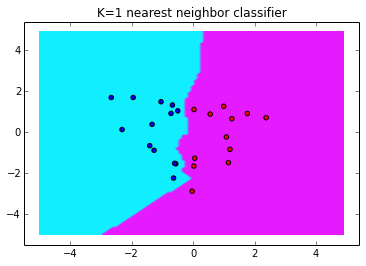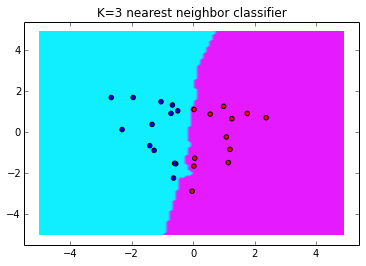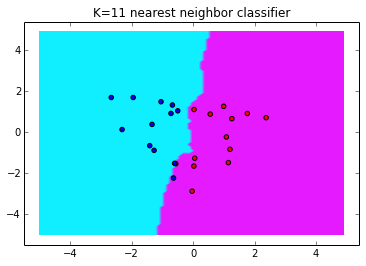That's neat. We can also have the model give us 'probabilities' - take the mean instead of the mode.

In :
#plot
plot_contour_scatter(model, 'K=1 nearest neighbor classifier', k=1, proba=True)
plot_contour_scatter(model, 'K=3 nearest neighbor classifier', k=3, proba=True)
plot_contour_scatter(model, 'K=11 nearest neighbor classifier', k=11, proba=True)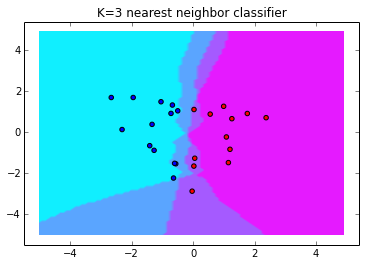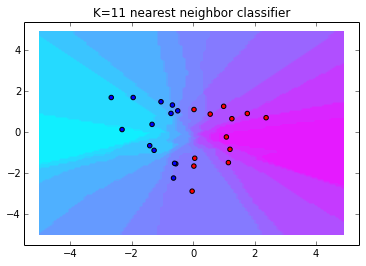Very interesting dynamics with the K=11 probabilistic classifier.

Another trick we can do is to get rid of the k parameter. We'll just have a kernel density estimator for each class and take the class with the highest estimated probability. In other words, we'll weight the predictions based on an exponential decay in distance.

In :
from scipy.stats import kde

class KernelDensityClassifier(object):

def __init__(self):
pass

def fit(self, X, y,k=0.5):
#build kernel density estimators
self.zero_kde = kde.gaussian_kde(X[where(y==0)].T, bw_method=k)
self.one_kde = kde.gaussian_kde(X[where(y==1)].T, bw_method=k)

def predict_proba(self, X, k=None):
#prediction based on kernel density
yhat = zeros(X.shape)
p_0 = self.zero_kde.evaluate(X.T).T
p_1 = self.one_kde.evaluate(X.T).T
#predict
return greater_equal(p_0,p_1)*(p_0) + less_equal(p_0,p_1)*(1-p_1)

def predict(self, X, k=3):
return around(self.predict_proba(X), decimals=0)


In :
for test in range(3):
X,y = make_classification(n_features=2, n_informative=2,
n_redundant=0, n_repeated=0, n_classes=2,
n_samples=50)

#train our model
model = KernelDensityClassifier()
model.fit(X,y)

#plot
plot_contour_scatter(model, 'Kernel Density Classifier', proba=True)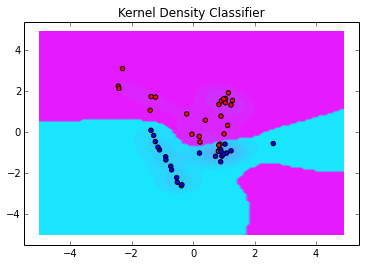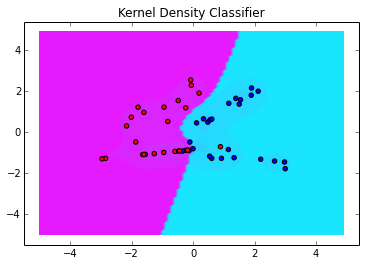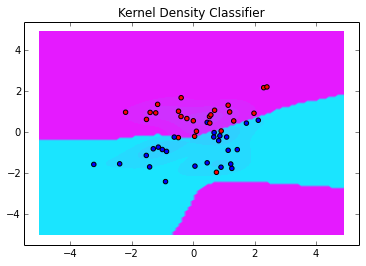The Kernel Density Classifier has similarities with RBF networks and SVM's with RBF kernels. It can be thought of as a RBF network with the centroids fixed on the training points, and the weights fixed based on class label.